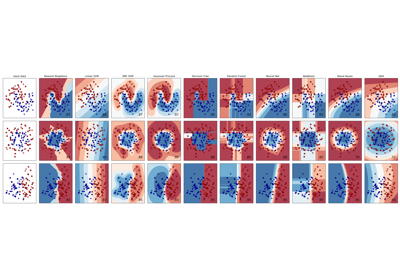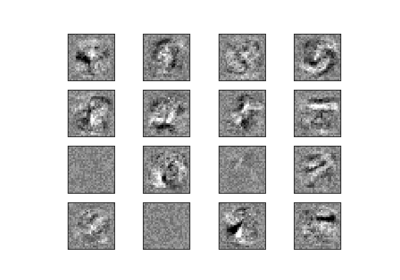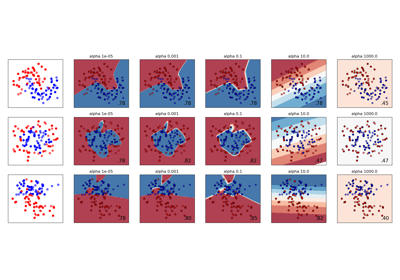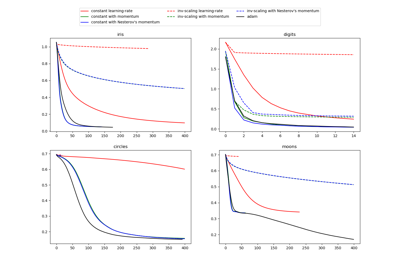sklearn.neural_network.MLPClassifier¶

class sklearn.neural_network.MLPClassifier(hidden_layer_sizes=(100, ), activation=’relu’, solver=’adam’, alpha=0.0001, batch_size=’auto’, learning_rate=’constant’, learning_rate_init=0.001, power_t=0.5, max_iter=200, shuffle=True, random_state=None, tol=0.0001, verbose=False, warm_start=False, momentum=0.9, nesterovs_momentum=True, early_stopping=False, validation_fraction=0.1, beta_1=0.9, beta_2=0.999, epsilon=1e-08, n_iter_no_change=10)[source]

Multi-layer Perceptron classifier.

This model optimizes the log-loss function using LBFGS or stochastic gradient descent.

New in version 0.18.

Notes

MLPClassifier trains iteratively since at each time step the partial derivatives of the loss function with respect to the model parameters are computed to update the parameters.

It can also have a regularization term added to the loss function that shrinks model parameters to prevent overfitting.

This implementation works with data represented as dense numpy arrays or sparse scipy arrays of floating point values.

References

Hinton, Geoffrey E.
“Connectionist learning procedures.” Artificial intelligence 40.1 (1989): 185-234.
Glorot, Xavier, and Yoshua Bengio. “Understanding the difficulty of
training deep feedforward neural networks.” International Conference on Artificial Intelligence and Statistics. 2010.
He, Kaiming, et al. “Delving deep into rectifiers: Surpassing human-level
performance on imagenet classification.” arXiv preprint arXiv:1502.01852 (2015).
Kingma, Diederik, and Jimmy Ba. “Adam: A method for stochastic
optimization.” arXiv preprint arXiv:1412.6980 (2014).

Methods

 fit(self, X, y) Fit the model to data matrix X and target(s) y. get_params(self[, deep]) Get parameters for this estimator. predict(self, X) Predict using the multi-layer perceptron classifier predict_log_proba(self, X) Return the log of probability estimates. predict_proba(self, X) Probability estimates. score(self, X, y[, sample_weight]) Returns the mean accuracy on the given test data and labels. set_params(self, \*\*params) Set the parameters of this estimator.
__init__(self, hidden_layer_sizes=(100, ), activation=’relu’, solver=’adam’, alpha=0.0001, batch_size=’auto’, learning_rate=’constant’, learning_rate_init=0.001, power_t=0.5, max_iter=200, shuffle=True, random_state=None, tol=0.0001, verbose=False, warm_start=False, momentum=0.9, nesterovs_momentum=True, early_stopping=False, validation_fraction=0.1, beta_1=0.9, beta_2=0.999, epsilon=1e-08, n_iter_no_change=10)[source]
fit(self, X, y)[source]

Fit the model to data matrix X and target(s) y.

Parameters: X : array-like or sparse matrix, shape (n_samples, n_features) The input data. y : array-like, shape (n_samples,) or (n_samples, n_outputs) The target values (class labels in classification, real numbers in regression). self : returns a trained MLP model.
get_params(self, deep=True)[source]

Get parameters for this estimator.

Parameters: deep : boolean, optional If True, will return the parameters for this estimator and contained subobjects that are estimators. params : mapping of string to any Parameter names mapped to their values.
partial_fit

Update the model with a single iteration over the given data.

Parameters: X : {array-like, sparse matrix}, shape (n_samples, n_features) The input data. y : array-like, shape (n_samples,) The target values. classes : array, shape (n_classes), default None Classes across all calls to partial_fit. Can be obtained via np.unique(y_all), where y_all is the target vector of the entire dataset. This argument is required for the first call to partial_fit and can be omitted in the subsequent calls. Note that y doesn’t need to contain all labels in classes. self : returns a trained MLP model.
predict(self, X)[source]

Predict using the multi-layer perceptron classifier

Parameters: X : {array-like, sparse matrix}, shape (n_samples, n_features) The input data. y : array-like, shape (n_samples,) or (n_samples, n_classes) The predicted classes.
predict_log_proba(self, X)[source]

Return the log of probability estimates.

Parameters: X : array-like, shape (n_samples, n_features) The input data. log_y_prob : array-like, shape (n_samples, n_classes) The predicted log-probability of the sample for each class in the model, where classes are ordered as they are in self.classes_. Equivalent to log(predict_proba(X))
predict_proba(self, X)[source]

Probability estimates.

Parameters: X : {array-like, sparse matrix}, shape (n_samples, n_features) The input data. y_prob : array-like, shape (n_samples, n_classes) The predicted probability of the sample for each class in the model, where classes are ordered as they are in self.classes_.
score(self, X, y, sample_weight=None)[source]

Returns the mean accuracy on the given test data and labels.

In multi-label classification, this is the subset accuracy which is a harsh metric since you require for each sample that each label set be correctly predicted.

Parameters: X : array-like, shape = (n_samples, n_features) Test samples. y : array-like, shape = (n_samples) or (n_samples, n_outputs) True labels for X. sample_weight : array-like, shape = [n_samples], optional Sample weights. score : float Mean accuracy of self.predict(X) wrt. y.
set_params(self, **params)[source]

Set the parameters of this estimator.

The method works on simple estimators as well as on nested objects (such as pipelines). The latter have parameters of the form <component>__<parameter> so that it’s possible to update each component of a nested object.

Returns: self

Examples using sklearn.neural_network.MLPClassifier¶Classifier comparisonVisualization of MLP weights on MNISTVarying regularization in Multi-layer PerceptronCompare Stochastic learning strategies for MLPClassifier